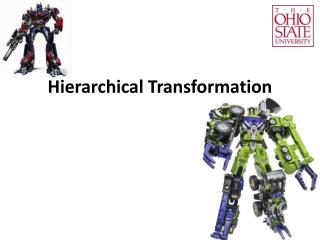DownloadDownload PresentationHierarchical Transformation

# Hierarchical Transformation

Download Presentation## Hierarchical Transformation

- - - - - - - - - - - - - - - - - - - - - - - - - - - E N D - - - - - - - - - - - - - - - - - - - - - - - - - - -
##### Presentation Transcript

1. Hierarchical Transformation

2. Summary • If we think: • Each transformation updates the vertex in an absolute world. • Then, the code is arranged in a reverse order. • Alternatively, OpenGL thinks: • A transformation updates the coordinate system. • For each change, the transformation is defined in the current (local) coordinate system. • Transformation matrix and coordinate system are equivalent. • The code is in the forward order!

3. A hierarchy glLoadIdentity(); //A dot at 0,0,0 W W

4. A hierarchy glLoadIdentity(); //A dot at 0,0,0 glTranslatef(2,0,0); //A dot at 0,0,0 W L1 W L1

5. A hierarchy glLoadIdentity(); //A dot at 0,0,0 glTranslatef(2,0,0); //A dot at 0,0,0 glTranslatef(1,1,0); glRotatef(45,0,0,1); //A dot at 0,0,0 W L1 L2 L2 W L1

6. A hierarchy glLoadIdentity(); //A dot at 0,0,0 glTranslatef(2,0,0); //A dot at 0,0,0 glTranslatef(1,1,0); glRotatef(45,0,0,1); //A dot at 0,0,0 glTranslatef(1,1,0); glRotate(45,0,0,1); //A dot at 0,0,0 W L1 L3 L2 L3 L2 W L1

7. A hierarchy L3 glLoadIdentity(); //A dot at 0,0,0 glTranslatef(2,0,0); //A dot at 0,0,0 glTranslatef(1.41,0,0); glRotatef(90,0,0,1); //A dot at 0,0,0 glTranslatef(1,1,0); glRotate(45,0,0,1); //A dot at 0,0,0 W L1 L2 L2 L3 W L1 Unchanged

8. A hierarchy W L1 L3 L4 L2 L3 L4 L2 • Draw in W • W->L1; Draw in L1 • L1->L2; Draw in L2 • L2->L3; Draw in L3 • L3->L2; • L2->L4; Draw in L4 W L1

9. A hierarchy W L1 L5 L2 L3 L4 L3 L4 • Draw in W • W->L1; Draw in L1 • L1->L2; Draw in L2 • L2->L3; Draw in L3 • L3->L2; • L2->L4; Draw in L4 • L4->L2 • L2->L1 • L1->L5; Draw in L5 L5 L2 W L1

10. Summary • The skeleton model can be defined as a tree. • Rendering can be done by depth-first transversal. • Matrix and coordinate system has the same idea: the matrix gives • You can use inverse transformation (not a good way) to rewind: • such as L3->L2, L2->L1… W L1 L5 L2 L3 L4

11. Matrix Stack //Transformation+Draw glPushMatrix(); //Transformation+Draw glPushMatrix(); //Transformation+Draw glPopMatrix(); //Transformation+Draw glPushMatrix(); //Transformation+Draw glPopMatrix(); //Transformation+Draw glPopMatrix(); Matrix 1 1 Matrix 2 2 1 Matrix 2 1 Matrix 3 3 1 Matrix 3 1 Matrix 1

12. Matrix Stack Instead of doing inverse transformation, we can easily rewind using the matrix stack. //Starts with W //Do W->L1 glPushMatrix(); //Back up L1 //Do L1->L2 glPushMatrix(); //Back up L2 //Do L2->L3 glPopMatrix(); //Restore L2 //Do L2->L4 glPopMatrix(); //Restore L1 //Do L1->L5 //Done W L1 L5 L2 L3 L4 Depth-First Traversal

13. The Human Body Hip Abdomen lButtock rButtock Chest lThigh rThigh Neck lCollar rCollar lShin rShin lFoot rFoot Head lShoulder rShoulder lToe rToe lForeArm rForeArm End End lHand rHand

14. Motion Capture

15. Performance Capture

16. BVH • The Biovision Hierarchy (BVH)file format was developed by Biovision, a defunct motion capture company. • It stores motion capture data. • It’s a text file. • It matches with OpenGL well. (That is why you will learn how to use it in Lab2.) • It has two parts: hierarchy and data.

17. Part 1: Hierarchy • The hierarchy is a joint tree. • Each joint has an offset and a channel list. • Offset is a bone vector (defined • in L1) from Joint 1 to Joint 2. • Let L12 be a shifted L1 at Joint 2. • The channel list defines a • sequence of transformations • from L12 to L2. L2 L12 Joint 2 L1 Joint 1

18. Part 1: Hierarchy • The channel list uses nine deformations: • Xposition, Yposition, Zposition • Xrotation, Yrotation, Zrotation • Xscale, Yscale, Zscale • The list only tells us transformation • types and their orders. The order is • the same as in OpenGL. • To know the actual transformation, • we need to read the data. L2 L12 Joint 2 L1 Joint 1

19. An example Chest Joint and its local coordinate system Neck Joint and its local coordinate system Neck’s offset from chest (like a bone) Channel list: Transformation from chest coordinate system to neck coordinate system

20. Part 2: Data Variable 1 Variable 2 Variable 3 Variable 4 Variable 5 Variable 6 Variable 7 Variable 8 Variable 9 • The data part stores a number of frames. • Each frame is a list of variables. • The model is animated, when each frame uses variables.

21. How to use BVH.h • BVH.h does most things for you. • Your job is to complete the render function. class BVH_NODE { public: char name; float offset; BVH_NODE* children; intchildren_number; float *tx, *ty, *tz; float *rx, *ry, *rz; float *sx, *sy, *sz; int data_order; } class BVH { public: BVH_NODE root; … void Read_File(…); void Set_Data(frame_id); void Forward(); void Backward(); void Render(); }

22. The Data Order In data_order: 1 means tx; 2 means ty; 3 means tz 4 means rx; 5 means ry; 6 means rz 7 means sx; 8 means sy; 9 means sz 0 means end • Translate *tz • Translate *ty • Scale *sx • Scale *sz • Rotate *ry • end 3 2 7 9 5 0

23. The Camera View gluLookAt(eye_x, eye_y, eye_z, center_x, center_y, center_z, up_x, up_y, up_z) • To change the camera view • We can update the gluLookAtfunction (which is not very easy…). • Or, we can combine gluLookAtwith transformation functions. (Check Lab2 for details.)